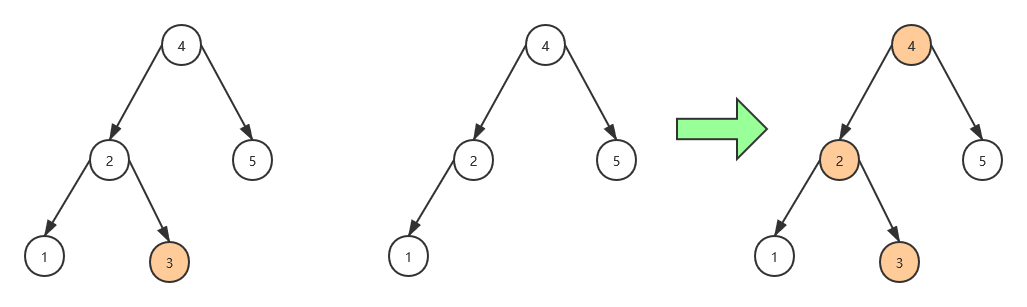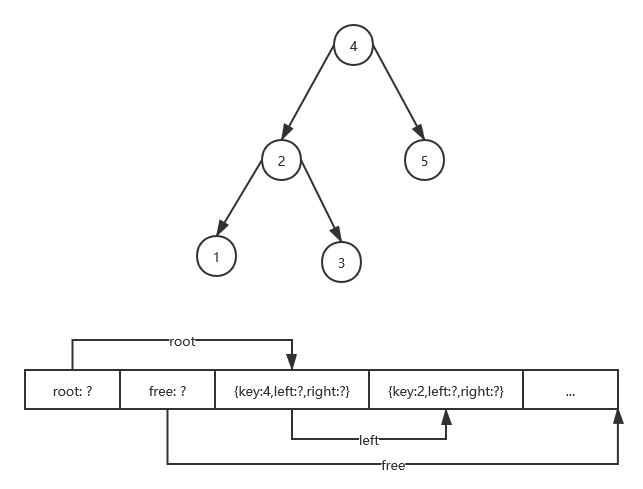# 重写 500 Lines or Less 项目 - DBDB

## 概述

• 允许快速访问任意用户设定的键
• 可包含海量数据————这意味着不能假定所有内容都位于内存中
• 基于不可变（immutable）数据的原则进行设计## 需求分析

### 基于不可变（immutable）数据的原则进行设计)

• 节点的键；
• 指向值的指针（必须存在）；
• 指向左节点的指针（若不存在则为0）；
• 指向右节点的指针（若不存在则为0）；

## 实现

### 步骤0：二叉树

``````class Node:
def __init__(self, key, value, left=None, right=None):
self.key = key
self.value = value
self.left = left
self.right = right
``````

``````class BinaryTree:
def __init__(self):
self._root = None

def get(self, key):
node = find(self._root, key)
return node.value

def set(self, key, value):
self._root = insert(self._root, key, value)

def delete(self, key):
self._root = remove(self._root, key)
``````

``````def find(node, key) -> Node:
if node is None:
elif node.key == key:
return node
elif key < node.key:
return find(node.left, key)
else:  # key > node.key
return find(node.right, key)
``````

``````def insert(node: Node, key, value) -> Node:
if not node:
return Node(key, value)
elif key < node.key:
return node.transform(left=insert(node.left, key, value))
elif key > node.key:
return node.transform(right=insert(node.right, key, value))
else:  # key == node.key
if node.value == value:
return node
return node.transform(value=value)
``````

``````class Node:
def transform(self, **kwargs):
return Node(self.key,
value=kwargs.get('value', self.value),
left=kwargs.get('left', self.left),
right=kwargs.get('right', self.right))
``````

• 删除叶子（末端）节点
• 删除只有左子树的节点
• 删除只有右子树的节点
• 删除左/右子树都存在的节点

### 步骤1：引用

``````MISSING = object()

class Ref:
self.target = target

def get(self):
assert self.target is not MISSING
return self.target

class ValueRef(Ref):
pass

class NodeRef(Ref):
pass
``````

`Ref` 可以接收两个参数：指向存储位置的指针以及实际值。这实际上包含了两种不同的访问方式：当新建节点时，我们需要指定值，但尚未确定存储地址；而从磁盘中加载时则采用延迟策略，只读取地址到内存中，后续真正访问值的时候再从地址加载数据。读者可能会注意到这里我添加了几个 `assert`，主要是因为两种不同的访问路径很容易误用，特别是对于 `Python` 这种缺乏编译时检查机制的语言，多做一些检查有助于尽早定位问题，免得错误扩散。

``````class Node:
def __init__(self, key, value_ref: ValueRef,
left_ref: NodeRef = None,
right_ref: NodeRef = None):
assert isinstance(value_ref, ValueRef)
assert left_ref is None or isinstance(left_ref, NodeRef)
assert right_ref is None or isinstance(right_ref, NodeRef)
self.key = key
self.value_ref = value_ref
self.left_ref = left_ref
self.right_ref = right_ref
``````

``````    @property
def value(self):
return self.value_ref.get()

@property
def left(self):
return NodeRef.get_node(self.left_ref)

@property
def right(self):
return NodeRef.get_node(self.right_ref)
``````

``````    def transform(self, **kwargs):
return Node(self.key,
value=kwargs.get('value', self.value),
left=kwargs.get('left', self.left),
right=kwargs.get('right', self.right))
``````

``````class Ref:
@classmethod
def transform(cls, current, data, key):
return cls(target=data[key]) if key in data else current

class Node:
def transform(self, **kwargs):
return Node(self.key,
value_ref=ValueRef.transform(self.value_ref, kwargs, 'value'),
left_ref=NodeRef.transform(self.left_ref, kwargs, 'left'),
right_ref=NodeRef.transform(self.right_ref, kwargs, 'right'))
``````

``````class Node:
@classmethod
def create(cls, key, value):
return cls(key=key,
value_ref=ValueRef(target=value))

def insert(node: Node, key, value) -> Node:
if not node:
return Node.create(key, value)
...
``````

### 步骤2：存储

• 它应该视为一个普通的字节流。在 `Python` 中对这种结构有一个很好的抽象：`File-like object`（以下简称 `FLO`）；
• 节点内容以数据块形式存储。具体存储格式需要我们自己确定，要简单的话，不妨使用语言内置的 `pickle` 机制（这也是本文采用的方法）。如果要方便调试，也可以考虑使用对人眼更加友好的格式，比如 `JSON`
• 它需要维护两个指针：指向根节点地址，以及指向可用存储位置的指针

``````class Storage:
def __init__(self, f, is_new):
pass  # TODO: to impl...

def memory() -> Storage:
return Storage(BytesIO(), is_new=True)

def file(file_name: str) -> Storage:
if os.path.exists(file_name):
mode, is_new = 'rb+', False
else:
mode, is_new = 'wb+', True
f = open(file_name, mode)
return Storage(f, is_new=is_new)
``````

``````class Storage:
def __init__(self, f, is_new: bool):
self._f = f
if is_new:
else:

def write_int(self, offset: int, value: int):
self.seek(offset)
self._f.write(struct.pack(INT_FORMAT, value))
``````

``````    def write_data(self, data) -> int:
pickle.dump(data, self._f)

``````

``````class BinaryTree:
def __init__(self, storage):
self._storage = storage
self._root_ref = None

@property
def root_node(self):
return NodeRef.get_node(self._root_ref)

@root_node.setter
def root_node(self, value):
if value == self.root_node:
return
self._root_ref = NodeRef(target=value) if value else None
``````

### 步骤3：序列化

``````    @property
def value(self):
return self.value_ref.get()
``````

``````class NodeManager(ABC):
@abstractmethod
pass

@abstractmethod
pass
``````

``````class Node:
"""Node of binary tree"""
def __init__(self, manager: NodeManager, key, value_ref: ValueRef,
left_ref: NodeRef = None,
right_ref: NodeRef = None):
assert isinstance(manager, NodeManager)
...
self.manager = manager
``````

``````    def get_child_node(self, ref: NodeRef):
if ref:
return ref.get()
return None

@property
def value(self):
return self.value_ref.get()

@property
def left(self):
return self.get_child_node(self.left_ref)

@property
def right(self):
return self.get_child_node(self.right_ref)
``````

``````class BinaryTree(NodeManager):
if ref.has_value():
return

if ref.has_value():
return
node = Node.deserialize(self, data)
ref.target = node
``````

``````class Node:
def serialize(self):
data = {
'key': self.key,
}
return data

def serialize_ref(self, data, key, ref: Ref):
if ref:

@classmethod
def deserialize(cls, manager, data):
key = data['key']
return Node(manager=manager,
key=key,
value_ref=value_ref,
left_ref=left_ref,
right_ref=right_ref)
``````

``````    def commit(self):
self.commit_node_ref(self._root_ref)
self._storage.flush()

def commit_node_ref(self, ref: NodeRef):
return
assert ref.has_value()
node = ref.get()
self.commit_value_ref(node.value_ref)
self.commit_node_ref(node.left_ref)
self.commit_node_ref(node.right_ref)

def commit_value_ref(self, ref: ValueRef):
assert ref and ref.has_value()
return
``````

### 步骤4：数据库接口

``````class DB:
def __init__(self, file_name: str):
self._storage = storage.file(file_name)
self._tree = BinaryTree(self._storage)

def close(self):
self._storage.close()

def __getitem__(self, key):
return self._tree.get(key)

def __setitem__(self, key, value):
self._tree.set(key, value)

def __delitem__(self, key):
self._tree.delete(key)

def commit(self):
self._tree.commit()
``````

• 读取头部信息（包括根节点和可用空间地址）；
• 读取根节点；

``````class Storage:
def __init__(self, f, is_new: bool):
...
self.locked = False

def lock(self):
if not self.locked:
portalocker.lock(self._f, portalocker.LOCK_EX)
self.locked = True

def unlock(self):
if self.locked:
portalocker.unlock(self._f)
self.locked = False
``````

`Storage` 只提供接口，至于加锁的时机则交给上层，也就是 `DB` 来控制：

``````    def reload_root(self):
self._storage.lock()
try:
finally:
self._storage.unlock()
``````

``````    def test_set_no_transaction(self):
self.db['k1'] = 'v1'
self.db['k2'] = 'v2'
self.assertEqual(2, self.db.commit_times)

def test_set_transaction(self):
with self.db.begin_transaction() as transaction:
self.db['k1'] = 'v1'
self.db['k2'] = 'v2'
transaction.commit()
self.assertEqual(1, self.db.commit_times)
``````

``````    def begin_transaction(self):
self.transaction = ManualTransaction(self)
return self.transaction

def get_current_transaction(self):
return self.transaction or auto_transaction(self)

def __setitem__(self, key, value):
with self.get_current_transaction():
self._tree.set(key, value)
``````

``````@contextlib.contextmanager
def auto_transaction(db):
yield
db.commit()
``````

``````class ManualTransaction:
def __init__(self, db):
self.db = db

def __enter__(self):
return self

def __exit__(self, exc_type, exc_val, exc_tb):
pass

def commit(self):
self.db.commit()
self.db.end_transaction()
``````

### 步骤5：命令行接口

``````def usage():
print('Usage:')
print('\t./cli.py <DBNAME> get <KEY>         - get value')
print('\t./cli.py <DBNAME> set <KEY> <VALUE> - set key value')
print('\t./cli.py <DBNAME> del <KEY>         - delete key')
print('\t./cli.py <DBNAME> purge             - delete database')
``````

``````def _get(db: DB, key):
print('{} => {}'.format(key, db[key]))

...

def main(argv):
try:
if not (2 <= len(argv) <= 4):
raise SyntaxError()
db_name, op = argv, argv
db = DB(db_name)
ops = {
'get': _get,
'set': _set,
'del': _del,
'purge': _purge,
}
if op not in ops:
raise SyntaxError()
ops[op](db, *argv[2:])
return 0
except (SyntaxError, TypeError):
usage()
return -1
``````